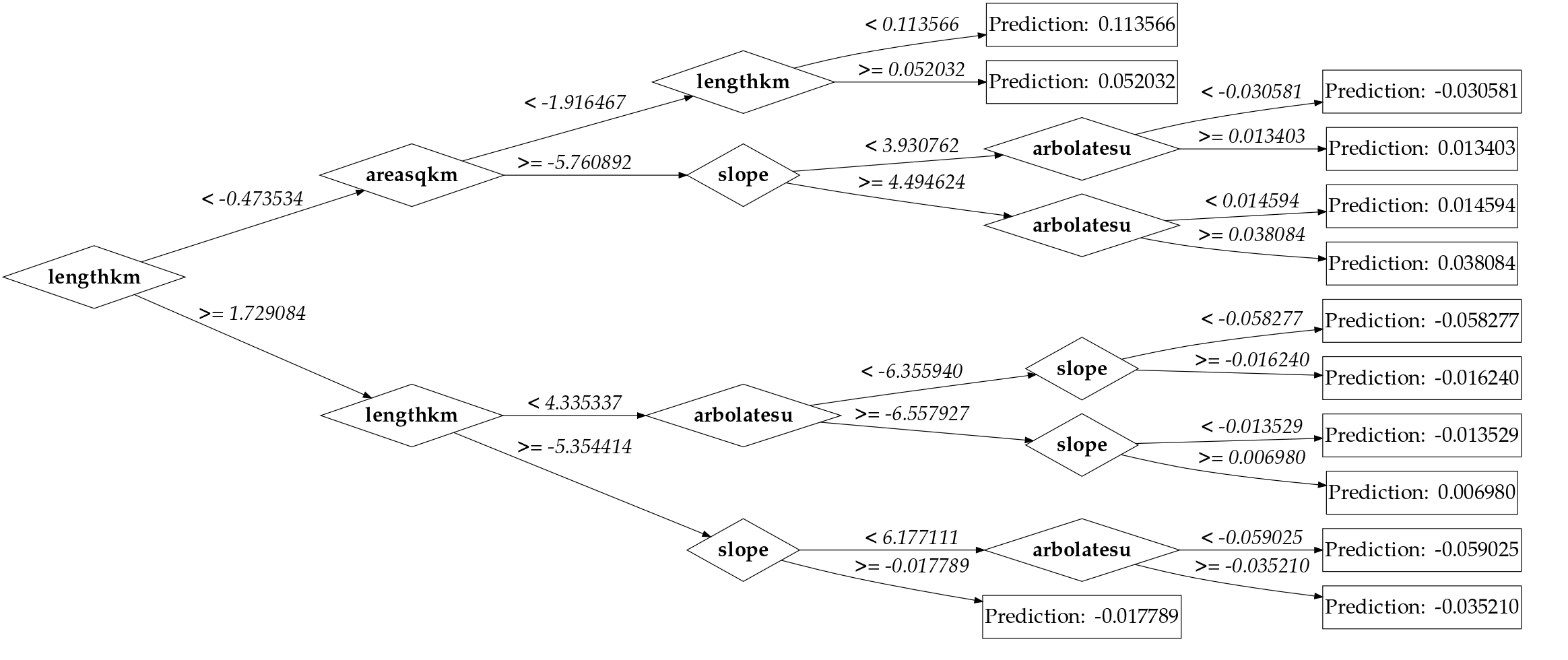While learning how to train Machine Learning models with the `caret` package in R for some research, I came across the need to visualizing iterations of a GBM model.

One of the resources I came across was this blog post by Gregory Kanevasky on plotting H20 decision trees in R. I wouldn’t say I copied some of it, but I definitely took a lot of inspiration from this post. The process itself involves taking your GBM model’s trees, and converting them to a `data.tree` object. Once they’re in the form of a `data.tree`, plotting is fairly simple – but if you’ve used the `gbm` package, attempting to plot the individual trees is not always exactly trivial.

So, I created a GitHub gist that provides the `build_tree()` function. Here is the code as of 12/4/2020:

``````
#' @title Build GBM Tree
#' @description Create a `data.tree` object from a GBM tree.
#' @param gbm_model Object of class `gbm`
#' @param i.tree Tree iteration to build from
#' @return A `data.tree` object from the `i.tree` tree of `gbm_model`.
build_tree <- function(gbm_model, i.tree = 1) {
gbm_tree <- gbm::pretty.gbm.tree(gbm_model, i.tree = i.tree)
pathString <- c("0" = "0")

for (node in seq(1, nrow(gbm_tree) - 1)) {
if (node %in% gbm_tree\$MissingNode[gbm_tree\$MissingNode != -1]) {
temp_string <- NA
# paste(
#     pathString[
#         which(
#             names(pathString) == as.character(
#                 which(gbm_tree\$MissingNode == node) - 1
#             )
#         )
#     ],
#     paste("(M)", node),
#     sep = "/"
# )
} else if (node %in% gbm_tree\$LeftNode[gbm_tree\$LeftNode != -1]) {
temp_string <- paste(
pathString[
which(
names(pathString) == as.character(
which(gbm_tree\$LeftNode == node) - 1
)
)
],
paste("(L)", node),
sep = "/"
)
} else if (node %in% gbm_tree\$RightNode[gbm_tree\$RightNode != -1]) {
temp_string <- paste(
pathString[
which(
names(pathString) == as.character(
which(gbm_tree\$RightNode == node) - 1
)
)
],
paste("(R)", node),
sep = "/"
)
}

pathString <- append(pathString, temp_string)
names(pathString) <- seq(0, length(pathString) - 1)
}

predictors <- gbm_model\$var.names
names(predictors) <- seq_len(length(predictors))
gbm_tree\$pathString <- unname(pathString)
gbm_data_tree <- data.tree::as.Node(gbm_tree)

# Plotting
data.tree::SetGraphStyle(gbm_data_tree, rankdir = "LR", dpi = 70)

data.tree::SetEdgeStyle(
gbm_data_tree,
fontname = "Palatino-italic",
labelfloat = TRUE,
fontsize = "26",
label = function(node) {
paste(
dplyr::if_else(grepl("(L)", node\$name, fixed = TRUE), "<", ">="),
formatC(as.numeric(node\$SplitCodePred), format = "f", digits = 6)
)
}
)

# Set node style for all of tree
data.tree::SetNodeStyle(
gbm_data_tree,
fontsize = "26",
fontname = function(node) dplyr::if_else(data.tree::isLeaf(node), "Palatino", "Palatino-bold"),
height = "0.75",
width = "1",
shape = function(node) dplyr::if_else(
data.tree::isLeaf(node),
"box",
"diamond"
),
label = function(node) dplyr::case_when(
data.tree::isLeaf(node) ~ paste("Prediction: ", formatC(as.numeric(node\$Prediction), format = "f", digits = 6)), # For leaves
node\$SplitVar == -1 ~ as.character(unname(predictors[as.character(gbm_tree\$SplitVar + 1)])), # For root node
TRUE ~ as.character(unname(predictors[as.character(node\$SplitVar + 1)])) # For every other node
)
)

gbm_data_tree
}
``````

Since the `data.tree` package allows us to build a `data.tree` object from a `pathString`, that’s simply all we need to do. However, while creating this by hand is simple, I wanted to create it programmatically. That’s where this loop comes in:

``````
for (node in seq(1, nrow(gbm_tree) - 1)) {
if (node %in% gbm_tree\$MissingNode[gbm_tree\$MissingNode != -1]) {
temp_string <- NA
# paste(
#     pathString[
#         which(
#             names(pathString) == as.character(
#                 which(gbm_tree\$MissingNode == node) - 1
#             )
#         )
#     ],
#     paste("(M)", node),
#     sep = "/"
# )
} else if (node %in% gbm_tree\$LeftNode[gbm_tree\$LeftNode != -1]) {
temp_string <- paste(
pathString[
which(
names(pathString) == as.character(
which(gbm_tree\$LeftNode == node) - 1
)
)
],
paste("(L)", node),
sep = "/"
)
} else if (node %in% gbm_tree\$RightNode[gbm_tree\$RightNode != -1]) {
temp_string <- paste(
pathString[
which(
names(pathString) == as.character(
which(gbm_tree\$RightNode == node) - 1
)
)
],
paste("(R)", node),
sep = "/"
)
}

pathString <- append(pathString, temp_string)
names(pathString) <- seq(0, length(pathString) - 1)
}
``````

Essentially, we create a named vector, `pathString`, and append to it depending on some conditions applied to each node. If the node is a left node, we attach (L) to the beginning of the node number. Likewise, if the node is a right node, we attach (R) to the beginning. If the node is instead a missing node, we do not want to add it to the tree. Once we’ve looped through every node in the tree, we un-name `pathString` and bind it to the gbm tree data frame. From this, we simply just call `data.tree::as.Node()` on it, and we have a `data.tree` gbm tree!

This gives us a tree that looks like:Note: this function is primarily for regression modeling. If you are training a classification model, you’ll need to adjust the `data.tree::SetEdgeStyle()` and `data.tree::SetNodeStyle()` parameters just slightly by removing `formatC()`. If your model is a mixed model, you can potentially use a vectorized `if else` (either the base package `ifelse()` or `dplyr::if_else()`).